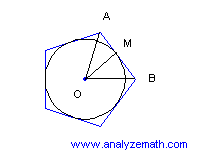# Polygons Problems

Regular polygons problems with detailed solutions.

## Problem 1

A 6 sided regular polygon (hexagon) is inscribed in a circle of radius 10 cm, find the length of one side of the hexagon.Solution to Problem 1:

• Angle AOB is given by
angle (AOB) = 360o / 6 = 60o
• Since OA = OB = 10 cm, triangle OAB is isosceles which gives
angle (OAB) = angle (OBA)
• So all three angles of the triangle are equal and therefore it is an equilateral triangle. Hence
AB = OA = OB = 10 cm.

## Problem 2

A circle of radius 6 cm is inscribed in a 5 sided regular polygon (pentagon), find the length of one side of the pentagon.(approximate your answer to two decimal places).Solution to Problem 2:
• Let t be the size of angle AOB, hence
t = 360o / 5 = 72o
• The polygon is regular and OA = OB. Let M be the midpoint of AB so that OM is perpendicular to AB. OM is the radius of the inscribed circle and is equal to 6 cm. Right angle trigonometry gives
tan(t / 2) = MB / OM
• The side of the pentagon is twice MB, hence
side of pentagon = 2 OM tan(t / 2) = 8.7 cm (answer rounded to two decimal places)

## Problem 3

Find the area of a dodecagon of side 6 mm. (approximate your answer to one decimal place).

Solution to Problem 3:

• A dodecagon is a regular polygon with 12 sides and the central angle t opposite one side of the polygon is given by.
t = 360o / 12 = 30o
• We now use the formula for the area when the side of the regular polygon is known
Area = (1 / 4) n x2 cot (180o / n)
• Set n = 12 and x = 6 mm
area = (1 / 4) (12) (6 mm)2 cot (180o / 12)
= 403.1 mm2 (approximated to 1 decimal place).

## Problem 4

Show that if the number of sides n of a polygon inscribed inside a circle of radius R, is very large then the area of the polygon may be approximated by the area of the circumscribed circle with radius R. (HINT: If angle x is very small and is in radians, then sin x may be approximated by x).

Solution to Problem 4:

• The area of a regular polygon with n sides may be given in terms of R by
area = (1/2) n R 2 sin (2 pi / n)
• If n is large, then 2 Pi /n is very small and sin (2 pi/n) may be approximated by 2 pi / n so that the area may be approximated by
area = (1/2) n R 2 (2 pi / n)
= pi R 2
• which is the area of the circle.
For more on the above question, see the interactive tutorial in regular polygons.

## More References and Links to Geometry Problems

Geometry Tutorials, Problems and Interactive Applets.